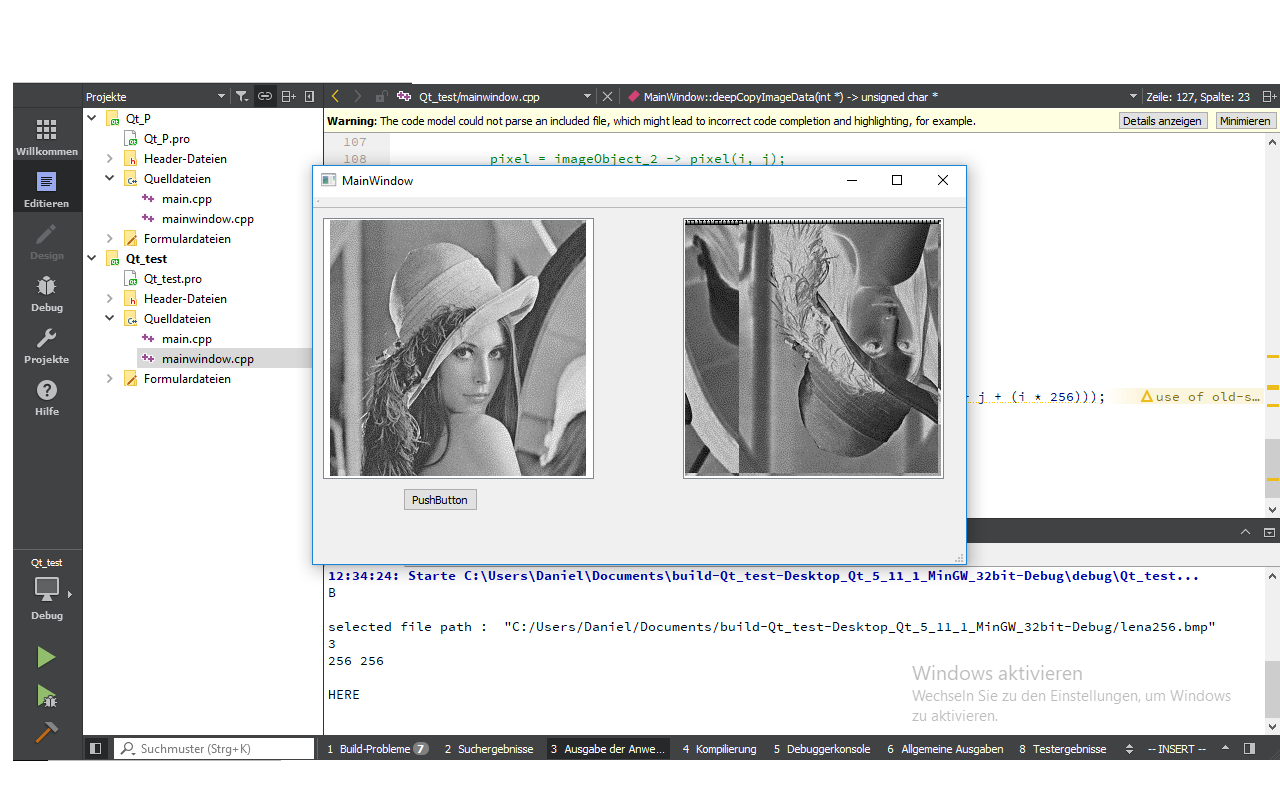# Convert QImage to char array and back

• Hello, I have to process images (.bmp and .png) in my GUI Qt project using a low-level programming language, which is C++ in my case. I'm not allowed to use any preconfigured methods who allow to process the image like OpenCV.
What is asked in the project:

• Enlarge, reduce image
• R.O.I
• image expansion
• image expansion using bilinear interpolation and spline (using polynomials)
• simple/multiple thresholding

I have already experience in processing an image in C using pointers and I would like to do the same in Qt.
I know to charge a QImage to an array of char (to edit it afterwards with pointers) but from this array of char BACK to the QImage I get an error message but ONLY when the image was edited using char *.
Here is my code (simplified)

``````unsigned char *temp;
QByteArray bytes;   //Fournie un tableau de type byte
QBuffer buffer(&bytes);
buffer.open(QIODevice::WriteOnly);

imageObject_2 = new QImage(*original_imageObject);

imageObject_2->save(&buffer, "BMP");
buffer.close();

unsigned char *data_image = (unsigned char *)malloc(bytes.size());
memcpy(data_image, reinterpret_cast<unsigned char *>(bytes.data()), (unsigned int)bytes.size());
*psize = bytes.size();

//Image processing using an algorithm

delete data_image;
data_image = nullptr;
image_2 = QPixmap::fromImage(*imageObject_2);
scene_2 = new QGraphicsScene(this);
scene_2 -> setSceneRect(image_2.rect());

ui -> graphicsView_2 -> setScene(scene_2);
``````

Can anyone explain why this doesn't work and how I can solve this problem.
Regards.

• Hi and welcome to devnet,

Why not use QImage's constructor that takes a char array as parameter ? That would be simpler.

By the way, what exact error are you getting ?

Also, there's no need to allocate QImage on the heap.

• Thank you very much, that worked out well.
But there is another problem, the image is incorrectly processed.

``````//Allocation of memory to edit image with unsigned char *
unsigned char *temp;
QByteArray bytes;   //Fournie un tableau de type byte
QBuffer buffer(&bytes);
buffer.open(QIODevice::WriteOnly);

QImage imageObject_2 = QImage(*original_imageObject);

imageObject_2.save(&buffer, "BMP");
buffer.close();

unsigned char *data_image = (unsigned char *)malloc(bytes.size());
memcpy(data_image, reinterpret_cast<unsigned char *>(bytes.data()), (unsigned int)bytes.size());
*psize = bytes.size();

//calculate negative of an image
unsigned char *val = data_image;
int i, j;
for(i = 0; i < imageObject_2.heigth(); i++)
{
for(j = 0; j < imageObject_2.width(); j++)
{
*(val + j + (i * 256)  = (uchar) (256 - *(val + j + (i * 256)));
}
}

//Creation QImage using raw data (unsigned char *)
QImage temp = QImage(data_image, imageObject_2.height, imageObject_2.width, QImage::Format_Indexed8); //Height and width are both 256, to find out the format I have used ImageObject_2.format()

//Convert image into Pixmap and print image
image_2 = QPixmap::fromImage(temp);
scene_2 = new GraphicsScene(this);
But the result is confusing as you can see in the added picture. The negative of the original image has been successfully calculated but it is been turned 180 degrees.!